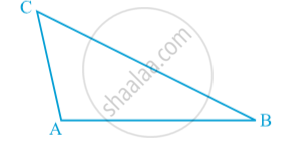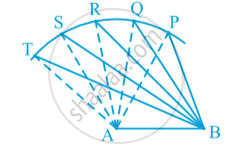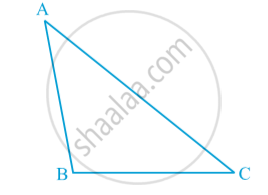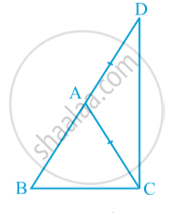Share

# Inequalities in a Triangle

#### text

Activity : Construct a scalene triangle (that is a triangle in which all sides are of different lengths). Measure the lengths of the sides. In ∆ ABC of following fig.BC is the longest side and AC is the shortest side. Also, ∠ A is the largest and ∠ B is the smallest.

#### theorem

Theorem : If two sides of a triangle are unequal, the angle opposite to the longer side is larger (or greater).
Activity :  Draw a line-segment AB. With A as centre and some radius, draw an arc and mark different points say P, Q, R, S, T  on it.  Join each of these points with A as well as with B in following fig .Observe that as we move from P to T, ∠ A is becoming larger and larger. The length of the side is also increasing; that is ∠ TAB > ∠ SAB > ∠ RAB > ∠ QAB > ∠ PAB and TB > SB > RB > QB > PB.
Now, draw any triangle with all angles unequal to each other. Measure the lengths of the sides in following fig.Observe that the side opposite to the largest angle is the longest. In Fig , ∠ B is the largest angle and AC is the longest side.

Theorem : In any triangle, the side opposite to the larger (greater)  angle is longer.
Now take a triangle ABC and in it, find AB + BC, BC + AC and AC + AB.
You will observe that AB + BC > AC, BC + AC > AB  and AC + AB > BC.

Theorem : The sum of any two sides of a triangle is greater than the third side.In above fig.  the side BA of ∆ ABC has been produced to a point D such that AD = AC.

### Shaalaa.com

Theorem : If two sides of a triangle are unequal, the angle opposite to the longer side is larger (or greater).. [00:08:56]
S
0%

S